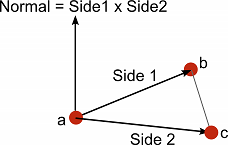Legacy Documentation: Version 4.6
Language: English
Direction and Distance from One Object to Another
The Amount of One Vector's Magnitude that Lies in Another Vector's Direction

# Computing a Normal/Perpendicular vector

Suggest a change

## Success!

Thank you for helping us improve the quality of Unity Documentation. Although we cannot accept all submissions, we do read each suggested change from our users and will make updates where applicable.

Close

## Sumbission failed

For some reason your suggested change could not be submitted. Please try again in a few minutes. And thank you for taking the time to help us improve the quality of Unity Documentation.

Close

Cancel

A normal vector (ie, a vector perpendicular to a plane) is required frequently during mesh generation and may also be useful in path following and other situations. Given three points in the plane, say the corner points of a mesh triangle, it is easy to find the normal. Pick any of the three points and then subtract it from each of the two other points separately to give two vectors:-``````var a: Vector3;
var b: Vector3;
var c: Vector3;

var side1: Vector3 = b - a;
var side2: Vector3 = c - a;

``````

The cross product of these two vectors will give a third vector which is perpendicular to the surface. The “left hand rule” can be used to decide the order in which the two vectors should be passed to the cross product function. As you look down at the top side of the surface (from which the normal will point outwards) the first vector should sweep around clockwise to the second:-

`````` var perp: Vector3 = Vector3.Cross(side1, side2);
``````

The result will point in exactly the opposite direction if the order of the input vectors is reversed.

For meshes, the normal vector must also be normalized. This can be done with the normalized property, but there is another trick which is occasionally useful. You can also normalize the perpendicular vector by dividing it by its magnitude:-

``````var perpLength = perp.magnitude;
perp /= perpLength;

``````

It turns out that the area of the triangle is equal to perpLength / 2. This is useful if you need to find the surface area of the whole mesh or want to choose triangles randomly with probability based on their relative areas.

Direction and Distance from One Object to Another
The Amount of One Vector's Magnitude that Lies in Another Vector's Direction